#### How to Count Unique Values in Excel

It is quite easy for you to get unique value where there are so many duplicates, especially if you would like to paste them in a new location. We are going to count unique values together.

Have a data with duplicates.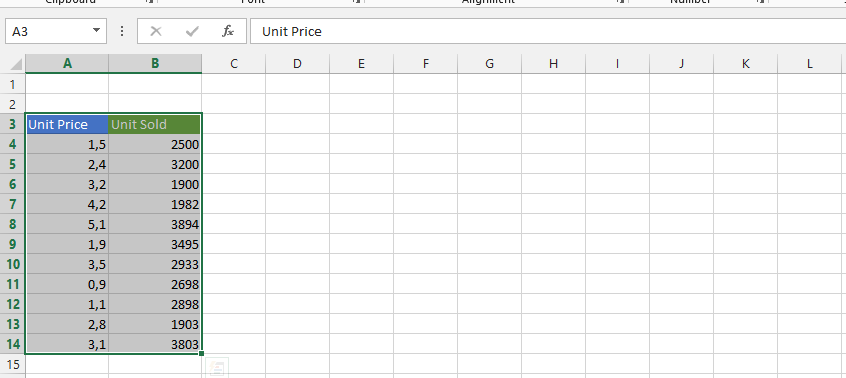Select the range of cells, or have it all in a table.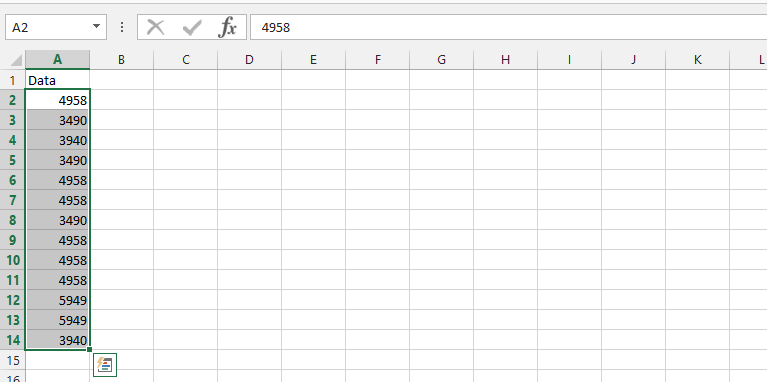Click on Data Tab (1), and choose Advanced (2) in Sort & Filter.Click yes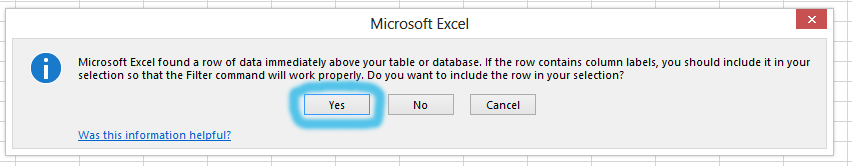Click copy to another location (1), where you want to copy it to (2), check on the unique records only (3), and click ok.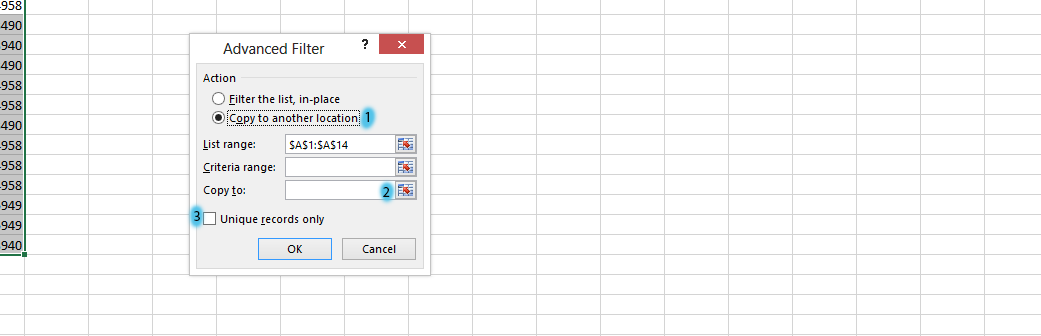List Range: =&A&1:&A&14

Note: If the cells you choose in number 2 isn’t large enough to contain all the unique numbers, Excel would automatically enlarge it.

Unique Values: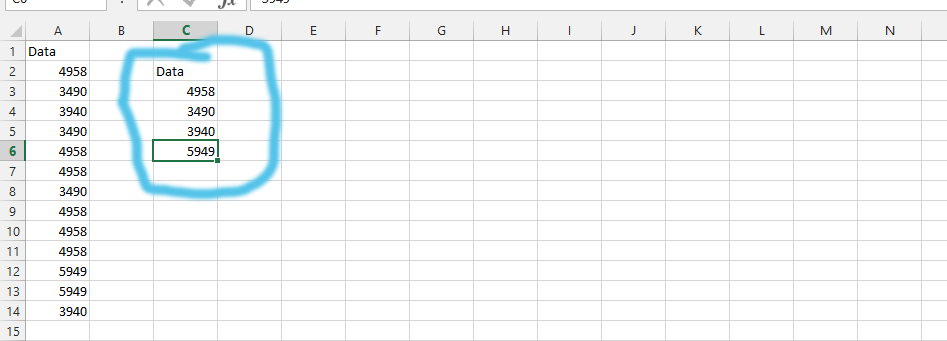## Sumproduct Formula

Alternative solution would be to use Sumproduct function combined with Countif function.

The formula to copy paste is: =SUMPRODUCT(1/COUNTIF(A2:A14,A2:A14)) where A2:A14 is the range of data set.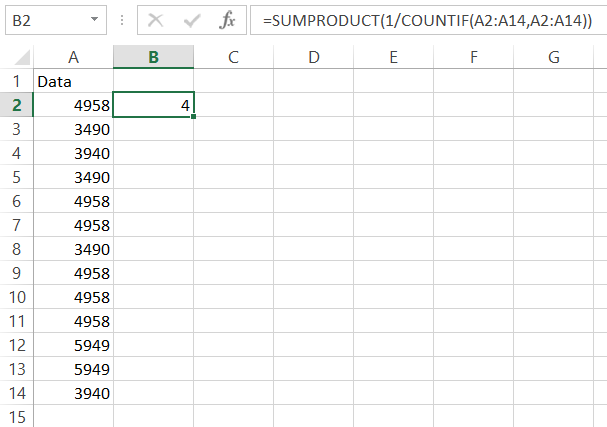Sum Formula

Another method to count unique values is a sum function combined with countif function again.

The formula is as follows: {=SUM(1/COUNTIF(A2:A14,A2:A14))}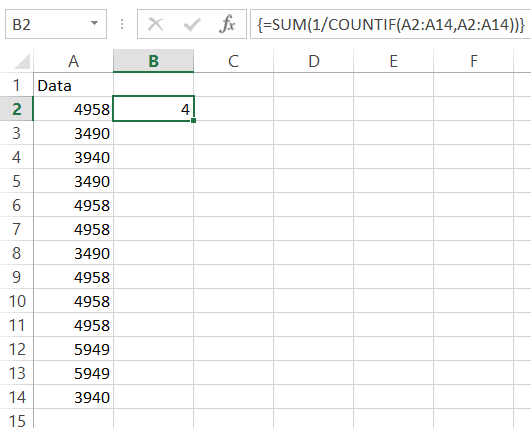For sure you have noticed curly braces. The formula is an array formula so to enter that you need to use CTRL + SHIFT + Enter buttons.

As you can see there is a possible that data in your tables or reports will be duplicated. To extract only disctinct/unique data like products/employee/companies/cities etc. just use this trick.

You can also count distinct values in pivot table.

## How to count unique values using Vba?

To count the number of unique values in a range using VBA in Microsoft Excel, you can use the following code:

`Function CountUnique(rng As Range) As Long`

`  Dim dict As Object `

`  Set dict = CreateObject("Scripting.Dictionary") `

`  For Each cell In rng `

`    dict(cell.Value) = 1 `

`  Next `

`  CountUnique = dict.Count `

`End Function `

To use this code, you need to follow these steps:

1. Open the Excel file that contains the data you want to count unique values for.
2. Press ALT + F11 to open the VBA editor.
3. In the editor, right-click on the workbook name in the Project Explorer and choose “Insert” and then “Module.”
4. Paste the code into the new module.